## The Calculator Encyclopedia Computes the Significant Figures of a Number

According to Wikipedia,

The significant figures (also known as the significant digits) of a number are digits that carry meaning contributing to its measurement resolution. This includes all digits except:

• Trailing zeros when they are merely placeholders to indicate the scale of the number (exact rules are explained at identifying significant figures); and
• Spurious digits introduced, for example, by calculations carried out to greater precision than that of the original data, or measurements reported to a greater precision than the equipment supports.

Nickzom Calculator computes the significant figures of any given number.

For Example: apply 3 significant figures to 123876  and apply 2 significant figures to 0.00009872.

First, you need to obtain the Nickzom Calculator – The Calculator Encyclopedia app.
You can get this app via any of these means:

Once, you have obtained the calculator encyclopedia app, proceed to the Calculator Map, then click on Significant Figures under the Mathematics section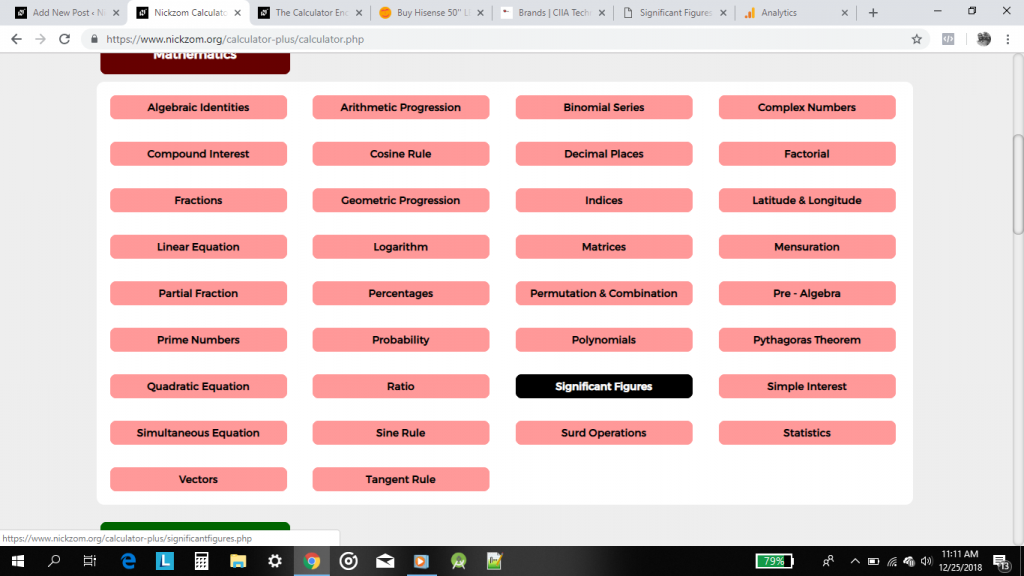On clicking the page or activity to enter the values is displayed.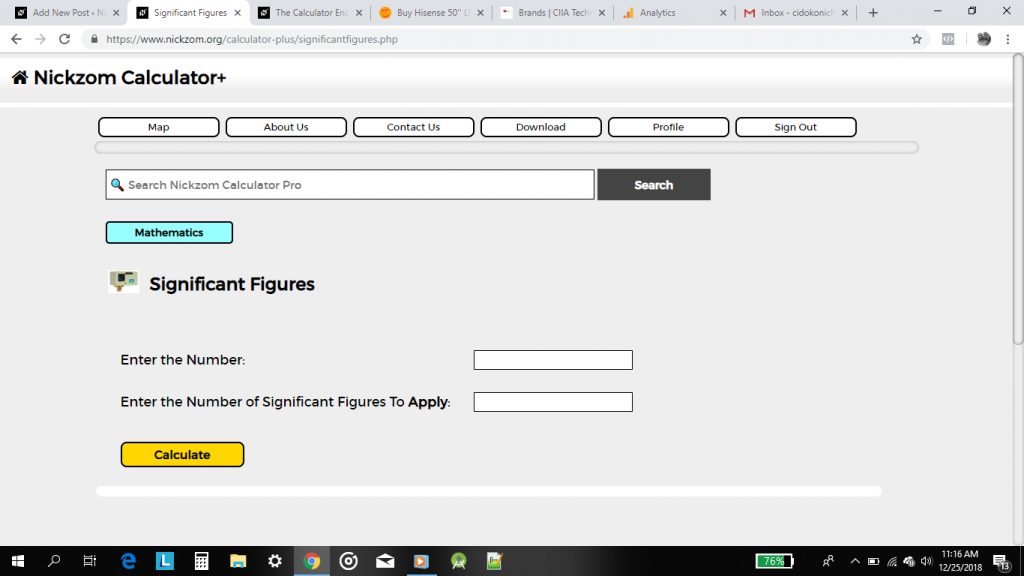From the first example: apply 3 significant figures to 123876
It implies that the number is 123876 and the number of significant figures to apply is 3.

## How to Solve and Calculate the Mean or Average of Discrete and Continuous Numbers

Mean is a measure of central tendency and is considered to be a very important parameter of statistics. Mean or Average is the sum of the data sets or numbers or values divided by the number of numbers or data sets or values.

What is a discrete number?

A discrete number is a standalone number. It might be a whole number or fractional number but it stands on its own with no extension or range. An example of a discrete number is 5, 12, 10.6, 17, 20

What is a continuous number?

A continuous number is a range of numbers packaged as a single entity. An example of a continuous number is 5 – 10, 20 – 30, 25 – 50.

There are two possibilities in calculating the mean of a set of discrete numbers. One can either compute the mean via the application of frequency or no frequency at all.

For Example: A set of discrete numbers such as these:

4, 5, 6, 7, 8, 9

These numbers all occur once and have a frequency of 1 per number.

Therefore, if you want to create a table for the number and frequency, it looks like this:

Number        4, 5, 6, 7, 8, 9

Frequency    1, 1, 1, 1, 1, 1

You can clearly see that there is no need for applying frequency to calculate the mean of the above set of numbers. Application of frequency on a large set of numbers makes it easier to organize and compute the mean.

Now, for a set of numbers such as these:

4, 4, 4, 2, 4, 5, 3, 3, 3, 2, 1, 1, 6, 4, 3, 2, 4, 2, 5, 2, 1

You can see that some of the discrete numbers occur more than once and this implies that application of frequency is useful and makes the computing of mean easier and comprehensive.

From the display of numbers above you can see that the number 4 occurred times, the number 2 occurred times, the number 5 occurred 2 times, the number 3 occurred 4 times, the number 1 occurred 3 times, the number 6 occurred 1 time.

## Mathematical Surd Operations can be Solved by Nickzom Calculator – The Calculator Encyclopedia

According to Laerd Mathematics,

Surds are numbers left in root form (√) to express its exact value. It has an infinite number of non-recurring decimals. Therefore, surds are irrational numbers.

According to Math is Fun,

• When it is a root and irrational, it is a surd
• Not all roots are surds

Nickzom Calculator solves calculations on surd and these calculations are categorized into:

• Surd Simplification A
• Surd Simplification B
• ‘Binary’ Surd Addition and Subtraction
• ‘Tenary’ Surd Addition and Subtraction
• Surd Multiplication
• Surd Division
• Surd Expansion
• Surd RationalizationNickzom makes use of a certain procedure to help the user easily input the surd values which is clearly represented in the image below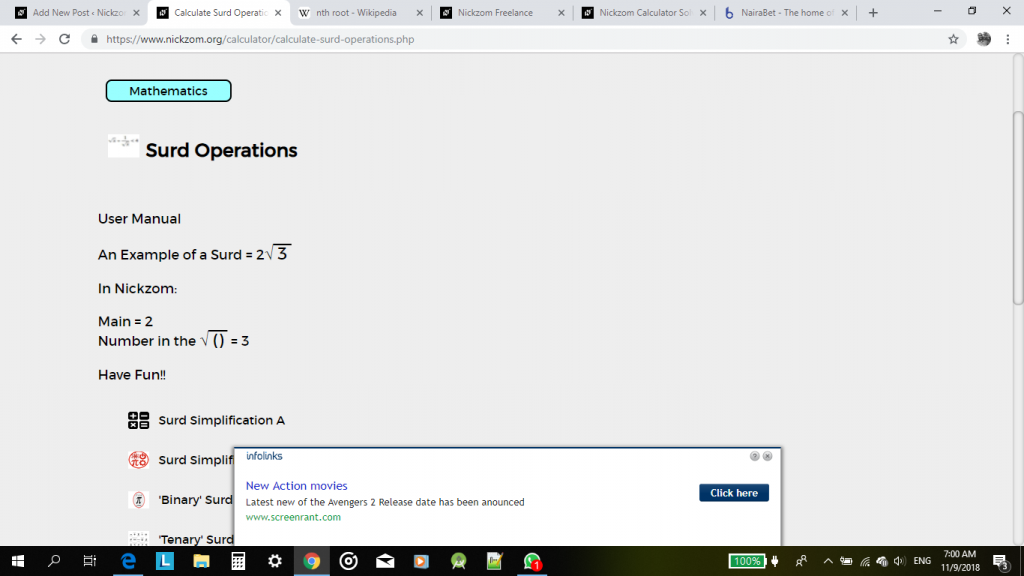For the purpose of this post, we would be handling a step by step guide on how to solve surd rationalization using Nickzom Calculator.

One can access Nickzom Calculator via any of these channels:

Now, click on Surd Rationalization, then enter the values appropriately using the user manual format.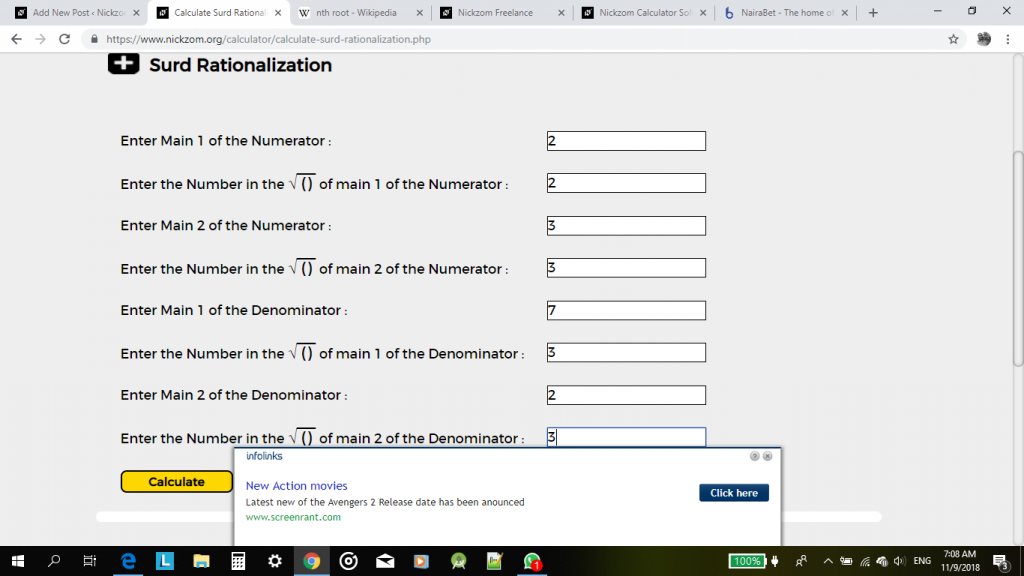Now, click on calculate to display the result.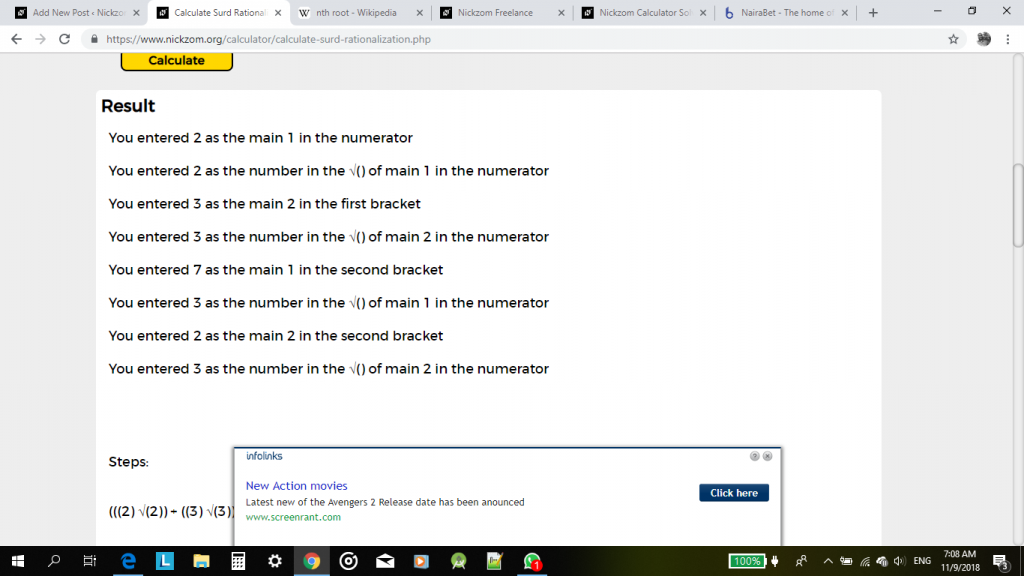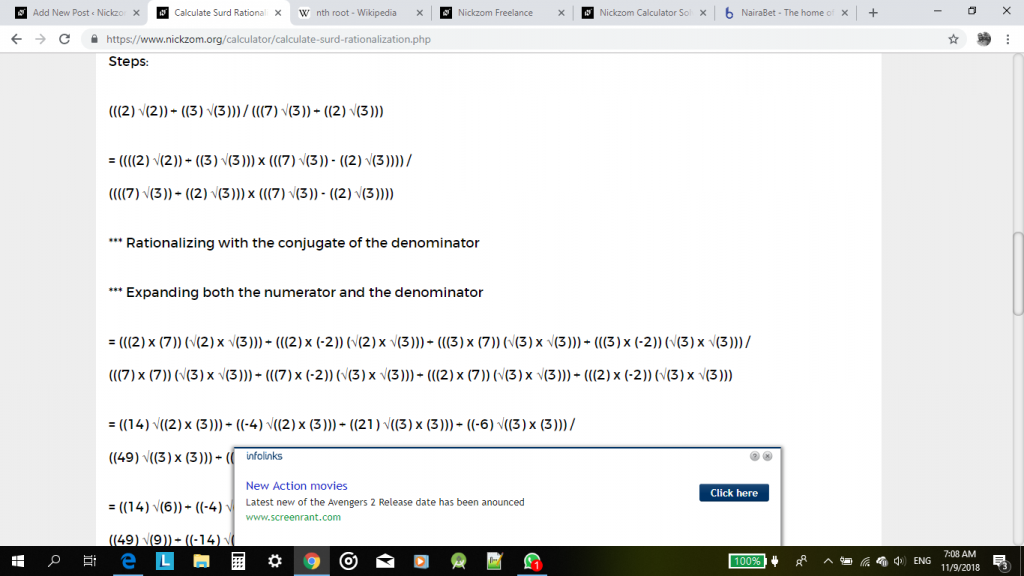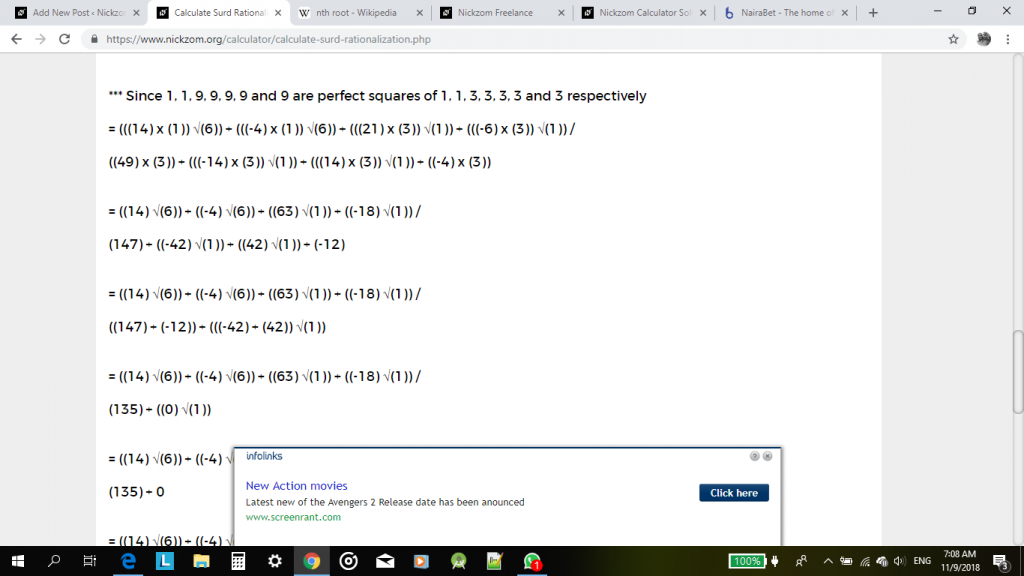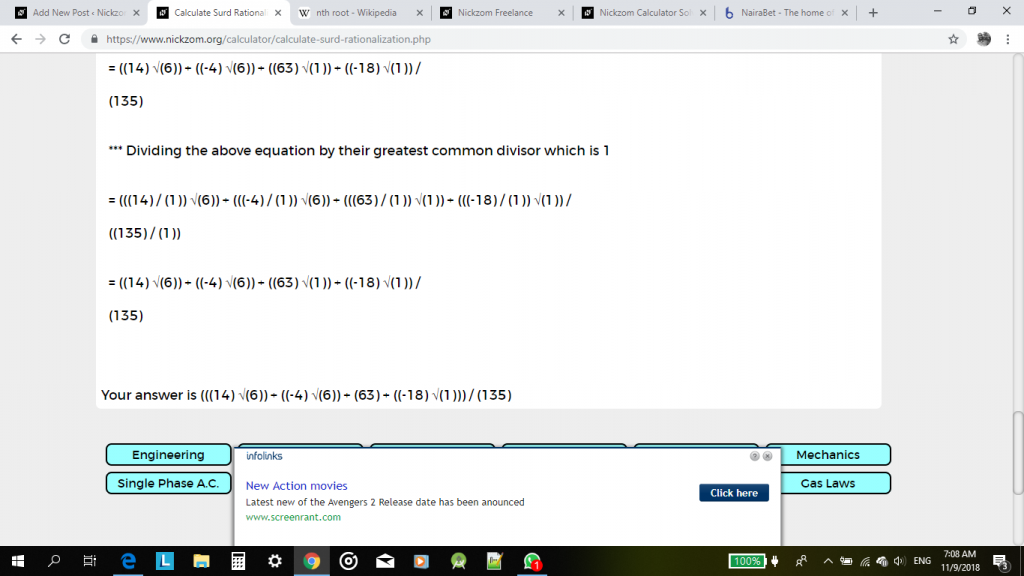I have seen that this steps and procedures might me cumbersome and a bit confusing for a reader or user. Therefore, an update would be coming soon with a new format of solving surd operations that can be easily understood and analysed. For now, with a bit of effort it could be understood or interpreted too.

Watch Out for the Update, Guys!